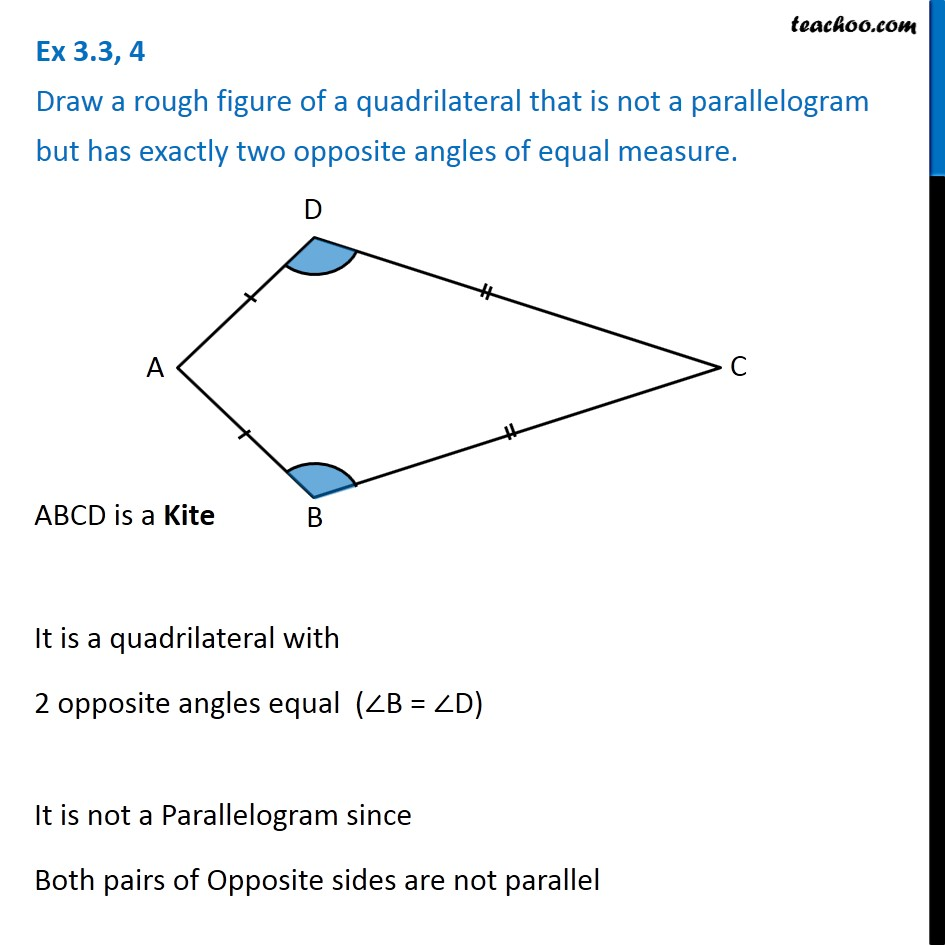Ex 3.3

Chapter 3 Class 8 Understanding Quadrilaterals
Serial order wiseLearn in your speed, with individual attention - Teachoo Maths 1-on-1 Class

### Transcript

Ex 3.3, 4 Draw a rough figure of a quadrilateral that is not a parallelogram but has exactly two opposite angles of equal measure. ABCD is a Kite It is a quadrilateral with 2 opposite angles equal (∠B = ∠D) It is not a Parallelogram since Both pairs of Opposite sides are not parallel